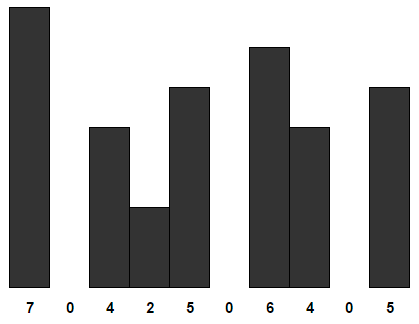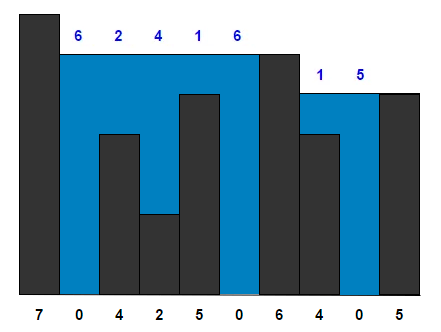# Trapping Rain Water within given set of bars

In trapping rain water problem, we need to find the maximum amount of water that can be trapped within given set of bars where width of each bar is 1 unit.

For example,

Input: Array containing height of bars {7, 0, 4, 2, 5, 0, 6, 4, 0, 5}Maximum amount of water that can be trapped is 25 as shown below (in blue color).The idea is to calculate maximum height bar on the left and right of every bar. Then the amount of water that can be stored on top of each bar is equal to minimum among maximum bar to the left and right minus height of current bar.

## C

Output:

Maximum amount of water that can be trapped is 25

## Java

Output:

Maximum amount of water that can be trapped is 25

The time complexity of above solution is O(n) and auxiliary space used by the program is O(n).

## C++

Output:

Maximum amount of water that can be trapped is 25

## Java

Output:

The time complexity of above solution is O(n) and auxiliary space used by the program is O(1).(1 votes, average: 5.00 out of 5)Loading...

Please use our online compiler to post code in comments. To contribute, get in touch with us.
Like us? Please spread the word and help us grow. Happy coding 🙂Subscribe
Notify ofGuest

Here’s another linear-time, constant space solution. Makes two passes, so it’s perhaps not as elegant as solution number 2 above, but it might be more intuitive to some.

Walk from left to right, keeping track of max as you go along. Any time the max changes, walk backward to the prior max, “filling with water” all intermediate bars.

Repeat, but from right to left.

Each bar is touched a constant number of times during each pass, once to compare against the max and once more to “fill with water.”

http://cpp.sh/5yf7spGuest

I think it needs three passes. What your algorithm return on {1,0,1}?Guest

It would be interesting to see this problem done an a 2 dimensional array.